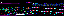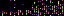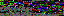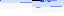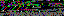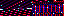```EVR.include("level/summary/graph/Bar.js");
EVR.Level.Summary.Graph.Plot = function(graph, sets)
{
this.graph = graph;
this.sets = sets;
this.plot();
}
EVR.Level.Summary.Graph.Plot.prototype.plot = function()
{
var graph = this.graph;
var sets = this.sets;
var set, cell, bar, bars = [];
for (var ii = 0; ii < sets.measurements.length; ii++)
{
for (var jj = 0; jj < sets.length; jj++)
{
set = sets[jj];
measurement = set.measurements[ii];
cell = graph.insert_plot_cell(jj == sets.length - 1);
if (ii == 0 && jj == 0)
{
}
bar = new EVR.Level.Summary.Graph.Bar(this, cell, measurement, set);
cell.appendChild(bar.element);
bars.push(bar);
}
}
this.bars = bars;
}
EVR.Level.Summary.Graph.Plot.prototype.draw = function()
{
var bars = this.bars;
for (var ii = 0; ii < bars.length; ii++)
{
bars[ii].draw();
}
}
EVR.Level.Summary.Graph.Plot.prototype.toString = function()
{
return "[object EVR.Level.Summary.Graph.Plot]";
}
```
```EVR.Level.Summary.Graph.Bar = function(plot, cell, measurement, set)
{
this.plot = plot;
this.cell = cell;
this.measurement = measurement;
this.set = set;
this.element = document.createElement("div");
this.style();
this.set_cell_height();
this.set_height();
}
EVR.Level.Summary.Graph.Bar.prototype.style = function()
{
var element = this.element;
element.style.width = GRAPH_BAR_WIDTH;
element.style.background = this.set.color;
element.style.fontSize = "0px";
}
EVR.Level.Summary.Graph.Bar.prototype.set_cell_height = function()
{
var height = GRAPH_PLOT_HEIGHT * this.plot.graph.field.get_dimensions();
this.cell_height = height;
this.cell.style.height = height;
}
EVR.Level.Summary.Graph.Bar.prototype.set_height = function()
{
var set = this.set;
var worst = set.worst;
var range = set.range;
var difference = Math.abs(this.measurement - worst);
var max = this.cell_height - GRAPH_PLOT_MARGIN;
var min = GRAPH_MIN_BAR_HEIGHT;
if (range == 0)
{
var height = max;
}
else
{
var height = ((max - min) * (difference / range)) + min;
}
this.element.style.height = height + "px";
}
EVR.Level.Summary.Graph.Bar.prototype.draw = function()
{
this.set_cell_height();
this.set_height();
}
EVR.Level.Summary.Graph.Bar.prototype.toString = function()
{
return "[object EVR.Level.Summary.Graph.Bar]";
}
```
```EVR.Level.Summary.Comparison.Model = function(comparison, color, text)
{
this.field = comparison.field;
this.color = color;
this.text = text;
this.cell = comparison.row.insertCell(-1);
this.cell.style.textAlign = "center";
this.set_proportions();
}
{
var text = this.text;
var family = MODEL_FONT_FAMILY;
var color = MODEL_FONT_COLOR;
var heading = new EVR.Text(this.cell, text, family, color, size, this.field);
var border = MODEL_BORDER;
style.borderRight = border;
style.borderBottom = border;
style.borderLeft = border;
}
{
var model = document.createElement("div");
var style = model.style;
var border = MODEL_BORDER;
style.background = this.color;
style.borderTop = border;
style.borderRight = border;
style.borderLeft = border;
this.cell.appendChild(model);
this.model = model;
}
EVR.Level.Summary.Comparison.Model.prototype.set_proportions = function()
{
var height = this.field.get_dimensions();
var model_height = height * MODEL_HEIGHT;
var style = this.model.style;
style.height = model_height;
style.width = model_height;
this.cell.style.width = model_height;
}
EVR.Level.Summary.Comparison.Model.prototype.draw = function()
{
this.set_proportions();
}
EVR.Level.Summary.Comparison.Model.prototype.toString = function()
{
return "[object EVR.Level.Summary.Comparison.Model]";
}
```
```EVR.include("level/summary/comparison/Model.js");
EVR.Level.Summary.Comparison = function(row, level)
{
this.row = row;
this.field = level.container;
}
{
var row = this.row;
var painter = this.painter;
var actual = painter.blend().get_string();
var expected = painter.blend(1).get_string();
var actual = new EVR.Level.Summary.Comparison.Model(
this, actual, MODEL_ACTUAL_TEXT);
var expected = new EVR.Level.Summary.Comparison.Model(
this, expected, MODEL_EXPECTED_TEXT);
this.models = [actual, expected];
}
EVR.Level.Summary.Comparison.prototype.draw = function()
{
var models = this.models;
for (var ii = 0; ii < models.length; ii++)
{
models[ii].draw();
}
}
EVR.Level.Summary.Comparison.prototype.toString = function()
{
return "[object EVR.Level.Summary.Comparison]";
}
```
```EVR.Level.Scoreboard.Speed = function()
{
this.sum = 0;
this.measurements = 0;
this.average = null;
}
{
this.sum += parseFloat(speed);
this.measurements++;
}
EVR.Level.Scoreboard.Speed.prototype.updateAverage = function()
{
this.average = this.sum / this.measurements;
}
EVR.Level.Scoreboard.Speed.prototype.getAverage = function()
{
return this.average;
}
```
35.172.223.30
35.172.223.30
35.172.223.30

November 9, 2013Food Spring - Watermelon Stage

Getting the fruit as far as possible is the object of each level, collecting bigger, more valuable guns. The final result is determined by the size of the fruits' collection when the monkey arrives in North America and either survives or perishes in the fruits' attack.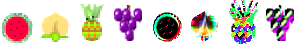Watermelon Peach Pineapple Grapes